### Prism:

Prism is uniform transparent medium bounded between two refracting surfaces, inclined at an angle.

### Refraction through a Prism:

A ray of light suffers two refractions on passing through a prism and hence deviates through a certain angle from its path.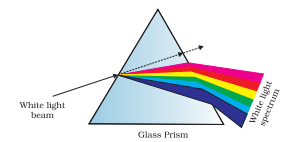### Angle of Deviation

The angle subtended between the direction of incident light ray and emergent light fay from a prism is called angle of deviation (δ).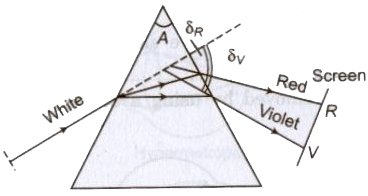### Prism Formula

The refractive index of material of prism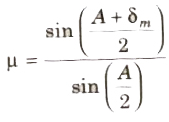### Dispersion of Light

The splitting of white light into its constituent colors in the sequence of VIBGYOR, on passing through a prism is called dispersion of light.

The refractive index μviolet > μRed, therefore, violet color deviates most and red color deviates least. i.e., δviolet > δRed.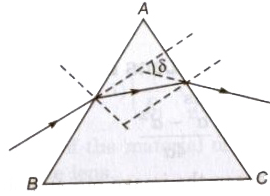### Angular Dispersion

The angle subtended between the direction of emergent violet and red rays of light from a prism is called angular dispersion.

Angular dispersion: Angular Dispersion produced by a prism for white light is the difference in the angles of deviation of 2 extreme colors i.e., violet & red colors.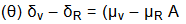where δv and δR are the angle of deviation.

### Dispersive Power:

The dispersive power of a prism is defined as the ratio of angular dispersion to the mean deviation produced y the prism. It is represented by W.where μY = (μ v + μ R ) / 2, is mean refractive index.

W depends only on nature of the material of prism while both mean & angular deviation depend on the angle of prism & material of the prism.